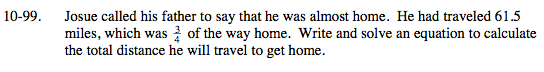### Home > MC2 > Chapter 10 > Lesson 10.2.2 > Problem10-99

10-99.Let x represent the total distance to home.

$\frac{3}{4}\; \text{of the total distance is 61.5 miles.}$

How can you represent this as an equation?

$\frac{3}{4}{\it x} = 61.5$

What does x equal?